# The velocity of a car on a circular path is shown. It starts at s = 0 and t = 0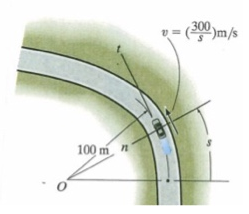The velocity of a car on a circular path is shown. It starts at s = 0 and t = 0. Determine its speed and acceleration magnitude at t 3s. Use n-t coordinates for this problem. (s in meters)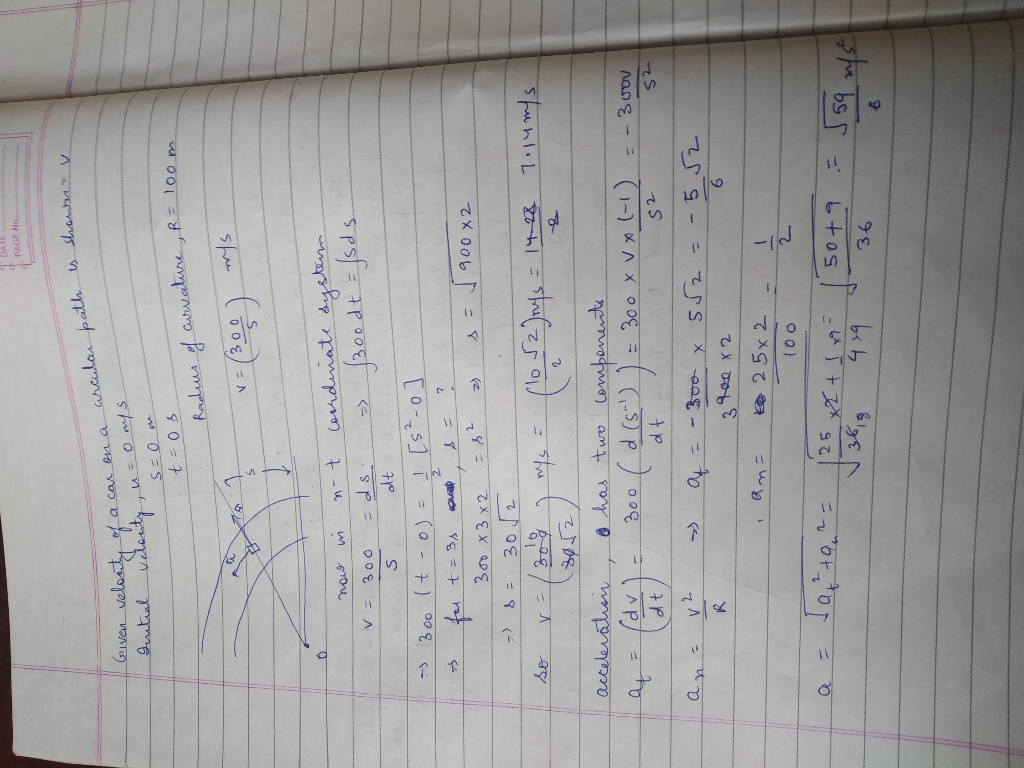#### Earn Coin

Coins can be redeemed for fabulous gifts.

Similar Homework Help Questions
• ### A car starts from rest and moves around a circular track of radius 32.0 m. Its...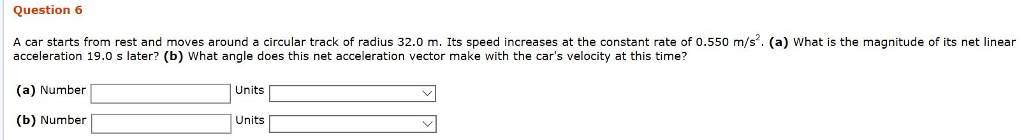A car starts from rest and moves around a circular track of radius 32.0 m. Its speed increases at the constant rate of 0.550 m/s2. (a) What is the magnitude of its net linear acceleration 19.0 s later? (b) What angle does this net acceleration vector make with the car's velocity at this time? Question 6 A car starts from rest and moves around a circular track of radius 32.0 m. Its speed increases at the constant rate of 0.550...

• ### Car B turns such that its speed is increased by (Q.)=(0.5e)m/s, where t is in seconds....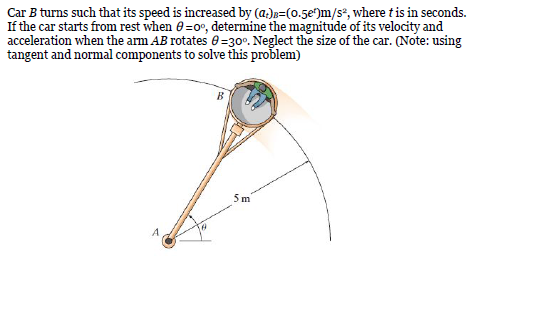Car B turns such that its speed is increased by (Q.)=(0.5e)m/s, where t is in seconds. If the car starts from rest when =0°, determine the magnitude of its velocity and acceleration when the arm AB rotates =30°. Neglect the size of the car. (Note: using tangent and normal components to solve this problem)

• ### A car initially traveling eastward turns north by traveling in a circular path at uniform speed...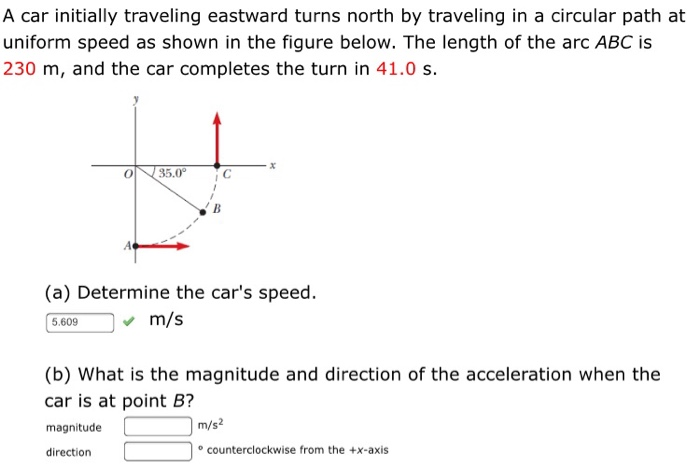A car initially traveling eastward turns north by traveling in a circular path at uniform speed as shown in the figure below. The length of the arc ABC is 230 m, and the car completes the turn in 41.0 s. (a) Determine the car's speed. 5.609m/s (b) What is the magnitude and direction of the acceleration when the car is at point B? magnitude m/s2 direction counterclockwise from the +x-axis

• ### The truck travels in a circular path having a radius of 50 m at a speed of 4 m/s. For a short...

The truck travels in a circular path having a radius of 50 m at a speed of 4 m/s. For a short distance from s = 0, its speed is increased by v = (0.05s) m/s2, where sis in meters. Determine the truck’s speed and magnitude of acceleration when it has moved s = 10 m.

• ### The boat is traveling along the circular path with a speed of v=(0.0625t2) m/s, where t...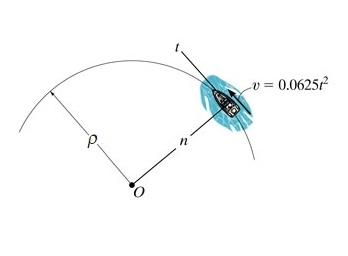The boat is traveling along the circular path with a speed of v=(0.0625t2) m/s, where t is in seconds. (Figure 1) Part A If ? = 42 m , determine the magnitude of its acceleration when t = 12 s . Express your answer to three significant figures and include the appropriate units.

• ### A stock car starts from rest at time t=0 with velocity (m/s) increasing for 3.7 s , according to the...

A stock car starts from rest at time t=0 with velocity (m/s) increasing for 3.7 s , according to the function vx=1.4t2+1.1t. Find the average acceleration for this interval

• ### A particle starts from rest at (0,0,0) at t=0 with velocity vector V=(3t2i-4tj+2t3k)m/s. 1) Find the...

A particle starts from rest at (0,0,0) at t=0 with velocity vector V=(3t2i-4tj+2t3k)m/s. 1) Find the velocity vector and acceleration vector at t=4s a) in rectangular coordinates b) in cylindrical coordinates c) in natural coordinates (n,t)

• ### A particle starts from the origin with velocity 3 m/s at t = 0 and moves in the xy plane with a...

A particle starts from the origin with velocity 3 m/s at t = 0 and moves in the xy plane with a varying acceleration given by= (8t),where is in meters per second squared and t is in seconds.(a) Determine the velocity of the particle as a function of time.

• ### A toy car starts at rest on a circular track of radius 2.30 m. If it...

A toy car starts at rest on a circular track of radius 2.30 m. If it uniformly increases speed to 1.70 m/s in 3.10 seconds, find the magnitude of the acceleration of the car at the very end of the trip.

• ### 2. The car starts from rest at s 0 and is subjected to an acceleration shown...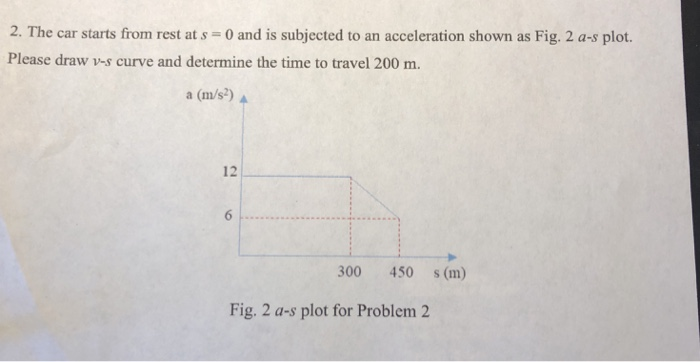2. The car starts from rest at s 0 and is subjected to an acceleration shown as Fig. 2 a-s plot. Please draw v-s curve and determine the time to travel 200 m. a (m/s) 수 12 300 450 s (m) Fig. 2 a-s plot for Problem 2